Also called Radau Quadrature (Chandrasekhar 1960). A Gaussian Quadrature with Weighting Functionin which the endpoints of the intervalare included in a total ofAbscissas, giving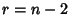free abscissas. Abscissas are symmetrical about the origin, and the general Formula is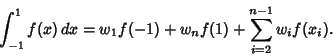(1)

The free Abscissasfor, ...,are the roots of the Polynomial, where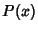is a Legendre Polynomial. The weights of the free abscissas are(2)(3)

and of the endpoints are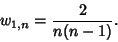(4)

The error term is given by(5)

for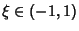. Beyer (1987) gives a table of parameters up to=11 and Chandrasekhar (1960) up to=9 (although Chandrasekhar's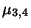foris incorrect).3 0 1.33333 ± 1 0.333333 4 ± 0.447214 0.833333 ± 1 0.166667 5 0 0.711111 ± 0.654654 0.544444 ± 1 0.100000 6 ± 0.285232 0.554858 ± 0.765055 0.378475 ± 1 0.0666667

The Abscissas and weights can be computed analytically for small.3 0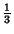4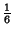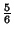5 0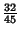References

Abramowitz, M. and Stegun, C. A. (Eds.). Handbook of Mathematical Functions with Formulas, Graphs, and Mathematical Tables, 9th printing. New York: Dover, pp. 888-890, 1972.

Beyer, W. H. CRC Standard Mathematical Tables, 28th ed. Boca Raton, FL: CRC Press, p. 465, 1987.

Chandrasekhar, S. Radiative Transfer. New York: Dover, pp. 63-64, 1960.

Hildebrand, F. B. Introduction to Numerical Analysis. New York: McGraw-Hill, pp. 343-345, 1956.